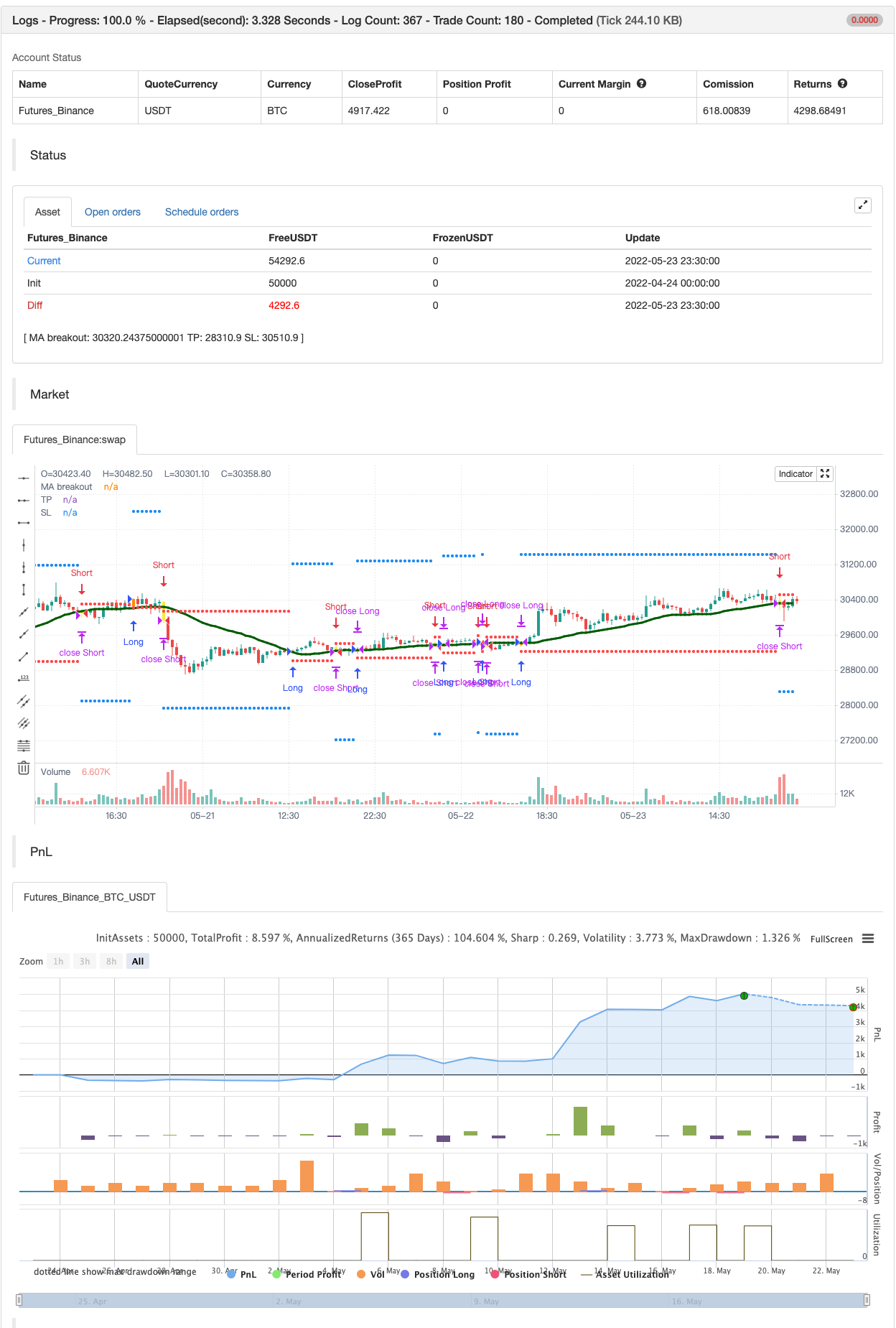# BEST Engulfing + Breakout Strategy

Author: 张超, Date: 2022-05-25 14:40:18
Tags: RMA EMA WMA

This is a simple algorithm for a Tradingview strategy tracking a convergence of 2 unrelated indicators.

Convergence is the solution to my trading problems. It’s a puzzle with infinite possibilities and only a few working combinations.

Here’s one that I like

• Engulfing pattern
• Price vs Moving average for detecting a breakout

Definition

Take out the notebooks :) and some coffee (good for focus). I’m bullish in coffee

The engulfing pattern is a two-candle reversal pattern. The second candle completely ‘engulfs’ the real body of the first one, without regard to the length of the tail shadows.

The bullish Engulfing pattern appears in a downtrend and is a combination of one red candle followed by a larger green candle The bearish Engulfing pattern appears in a downtrend and is a combination of one green candle followed by a larger red candle

Example: https://imgur.com/a/krDDUz4

We’re bored sir… what’s the point of all this?

In summary, an engulfing is a pattern to track reversals. (the whole TradingView audience stands up now giving a standing ovation) Adding the Price vs Moving average filters allows to track reversals with momentums (half of the audience collapsed because this is too awesome)

Ok sir… you picked up my interest

I included some cool backtest filters:

• date range filtering
• flexible take profit in USD value (plotted in blue)
• flexible stop loss in USD value (plotted in red)

All the best Dave

backtest```/*backtest
start: 2022-04-24 00:00:00
end: 2022-05-23 23:59:00
period: 30m
basePeriod: 15m
exchanges: [{"eid":"Futures_Binance","currency":"BTC_USDT"}]
*/

//@version=4
//@author=Daveatt

StrategyName = "BEST Engulfing + MA"
ShortStrategyName = "BEST Engulfing + MA"

strategy(title=StrategyName, shorttitle=ShortStrategyName, overlay=true,
pyramiding=2, default_qty_value=500, precision=7, currency=currency.USD,
commission_value=0.2,commission_type=strategy.commission.percent, initial_capital=10000)

includeEngulfing = true

includeMA = true
source_ma = input(title="Source Price vs MA", type=input.source, defval=close)
typeofMA = input(title="Type of MA", defval="SMA", options=["RMA", "SMA", "EMA", "WMA", "VWMA", "SMMA", "KMA", "TMA", "HullMA", "DEMA", "TEMA"])
length_ma = input(32, title = "MA Length", type=input.integer)

// ---------- Candle components and states
GreenCandle = close > open
RedCandle = close < open
NoBody = close==open
Body = abs(close-open)

// bullish conditions
isBullishEngulfing1 = max(close,open) < max(close,open) and min(close,open) > min(close,open) and Body > Body and GreenCandle and RedCandle
isBullishEngulfing2 = max(close,open) < max(close,open) and min(close,open) <= min(close,open) and Body > Body and GreenCandle and RedCandle

// bearish conditions
isBearishEngulfing1 = max(close,open) < max(close,open) and min(close,open) > min(close,open) and Body > Body and RedCandle and GreenCandle
isBearishEngulfing2 = max(close,open) >= max(close,open) and min(close,open) > min(close,open) and Body > Body and RedCandle and GreenCandle

// consolidation of conditions
isBullishEngulfing = isBullishEngulfing1 or isBullishEngulfing2
isBearishEngulfing = isBearishEngulfing1 or isBearishEngulfing2

//isBullishEngulfing = max(close,open) < max(close,open) and min(close,open) > min(close,open) and Body > Body and GreenCandle and RedCandle
//isBearishEngulfing = max(close,open) < max(close,open) and min(close,open) > min(close,open) and Body > Body and RedCandle and GreenCandle

Engulf_Buy = Engulf_curr < 0 ? 1 : 0
Engulf_Sell = Engulf_curr > 0 ? 1 : 0

// Price vs MM

smma(src, len) =>
smma = 0.0
smma := na(smma) ? sma(src, len) : (smma * (len - 1) + src) / len
smma

ma(smoothing, src, length) =>
if smoothing == "RMA"
rma(src, length)
else
if smoothing == "SMA"
sma(src, length)
else
if smoothing == "EMA"
ema(src, length)
else
if smoothing == "WMA"
wma(src, length)
else
if smoothing == "VWMA"
vwma(src, length)
else
if smoothing == "SMMA"
smma(src, length)
else
if smoothing == "HullMA"
wma(2 * wma(src, length / 2) - wma(src, length), round(sqrt(length)))
else
if smoothing == "LSMA"
src
else
if smoothing == "KMA"
xPrice = src
xvnoise = abs(xPrice - xPrice)
nfastend = 0.666
nslowend = 0.0645
nsignal = abs(xPrice - xPrice[length])
nnoise = sum(xvnoise, length)
nefratio = iff(nnoise != 0, nsignal / nnoise, 0)
nsmooth = pow(nefratio * (nfastend - nslowend) + nslowend, 2)
nAMA = 0.0
nAMA := nz(nAMA) + nsmooth * (xPrice - nz(nAMA))
nAMA
else
if smoothing == "TMA"
sma(sma(close, length), length)
else
if smoothing == "DEMA"
2 * src - ema(src, length)
else
if smoothing == "TEMA"
3 * (src - ema(src, length)) + ema(ema(src, length), length)
else
src

MA = ma(typeofMA, source_ma, length_ma)

plot(MA, color=#006400FF, title="MA breakout", linewidth=3)

macrossover  = crossover (source_ma, MA)
macrossunder = crossunder(source_ma, MA)

macross_curr = 0 - since_ma_sell + since_ma_buy
bullish_MA_cond = macross_curr < 0 ?  1 : 0
bearish_MA_cond = macross_curr > 0 ? 1  : 0

posUp = (Engulf_Buy ? 1 : 0) + (bullish_MA_cond ? 1 : 0)
posDn = (Engulf_Sell ? 1 : 0) + (bearish_MA_cond ? 1 : 0)

conditionUP = posUp == 2 and posUp < 2
conditionDN = posDn == 2 and posDn < 2

// primary-first signal of the trend
nUP = crossunder(sinceUP,sinceDN)
nDN = crossover(sinceUP,sinceDN)

// and the following secondary signals

// save of the primary signal

sell_trend  = sinceNDN < sinceNUP

// engulfing by
barcolor(nUP ? color.orange : na, title="Bullish condition")
barcolor(nDN ? color.yellow : na, title="Bearish condition")

isLong  = nUP
isShort = nDN

long_entry_price    = valuewhen(nUP, close, 0)
short_entry_price   = valuewhen(nDN, close, 0)

longClose   = close < MA
shortClose  = close > MA

///////////////////////////////////////////////
//* Backtesting Period Selector | Component *//
///////////////////////////////////////////////

StartYear = input(2017, "Backtest Start Year",minval=1980)
StartMonth = input(1, "Backtest Start Month",minval=1,maxval=12)
StartDay = input(1, "Backtest Start Day",minval=1,maxval=31)
testPeriodStart = timestamp(StartYear,StartMonth,StartDay,0,0)

StopYear = input(2020, "Backtest Stop Year",minval=1980)
StopMonth = input(12, "Backtest Stop Month",minval=1,maxval=12)
StopDay = input(31, "Backtest Stop Day",minval=1,maxval=31)
testPeriodStop = timestamp(StopYear,StopMonth,StopDay,0,0)

testPeriod() => true

//////////////////////////
//* Profit Component *//
//////////////////////////

input_tp_pips = input(2000, "Backtest Profit Goal (in USD)",minval=0)
input_sl_pips = input(200, "Backtest STOP Goal (in USD)",minval=0)

tp = buy_trend? long_entry_price + input_tp_pips : short_entry_price - input_tp_pips
sl = buy_trend? long_entry_price - input_sl_pips : short_entry_price + input_sl_pips

long_TP_exit  = buy_trend and high >= tp
short_TP_exit = sell_trend and low <= tp

plot(tp, title="TP", style=plot.style_circles, linewidth=3, color=color.blue)
plot(sl, title="SL", style=plot.style_circles, linewidth=3, color=color.red)

if testPeriod()
strategy.entry("Long", 1, when=isLong)
strategy.close("Long", when=longClose )